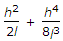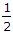# Civil Engineering - Surveying - Discussion

Discussion Forum : Surveying - Section 1 (Q.No. 2)
2.
If h is the difference in level between end points separated by l, then the slope correction is. The second term may be neglected if the value of h in a 20 m distance is less thanm
1 m
2 m
3 m
Explanation:
No answer description is available. Let's discuss.
Discussion:
66 comments Page 1 of 7.

Chaan A said:   3 months ago
Explain it clearly.

Second term will be neglected when h=3cm not 3meter for length 20 meter.

Mukesh das said:   2 years ago
How it will be 3m?

Please explain clearly with the completed formula.

Mukesh das said:   2 years ago
How it will be 3m?

Please explain clearly with the completed formula.
(1)

Dev Narayan Yadav said:   2 years ago
l = 20 m.
H2 = 2l,
H2 = 2*20,
H2 = 40.
And;
H = 6.32 or 6.33,
√40 = 6.32.

Khagendra said:   2 years ago
For slope (M) up to 20%.
Slope correction(Sc) = h^2/(2l).
Here d = 20m,
M = h/d,
0.2 = h/20.
H = 0.2 * 20 = 4m.
Here the nearest one is 3m.
So 3m is the appropriate answer.
(2)

Kartabo Ketema said:   3 years ago
How it will be 3m? Explain it with the formula.

Nandhu said:   3 years ago
The second term can be negleted only if the slopes flatter than 1 in 25..
That means 4%.

i.e, 4% of 20m = 0.8(4/5) ie, H shouldn't higher than 0.8m.
So option A is right.
(1)

Fayez abusafaqa ps said:   3 years ago
The error can be accepted upto 10%. thus, the second term divided by the correlational factor equal 0.1.

Assuming the first term is x and the second term is y. Then y/x+y=0.1==> x+y/x=10 with y not zero==>1+x/y=9==>y=9. solve it u will get l=3.

Logeshkanna said:   3 years ago
The answer should be A. 1/2m.

With ref of Punmia : the second term in slope correction shall be neglected for slopes flatter than about 1 in 25 (i.e slope should be lesser than 0.04).

The slope is Vertical distance/Horizontal distance.
.
Here Vertical distance is h and the horizondal distance is √((L)^2 - (h)^2).

L = 20 given in the problem. We need to find, for which value of h the slope will be less than 0.04.

Sub h = 0.5, we get slope = 0.025;
Sub h = 1, we get slope = 0.050;
Sub h = 2, we get slope = 0.100;
Sub h = 3, we get slope = 0.150;

Therefore Option A (h=0.5m) is the correct answer.